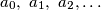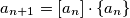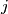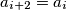### IMO Shortlist 2006 problem A1

Kvaliteta:
Avg: 3,0
Težina:
Avg: 6,0$a_{0},\ a_{1},\ a_{2},\dots$ is a sequence of real numbers such thatprove that exist$j$ such that for every$i\geq j$ we have$a_{i + 2} = a_{i}$.
Izvor: Međunarodna matematička olimpijada, shortlist 2006